# Chebyshev’s Bias: Chart for DELTA{24,23,1}(x)

We are publishing the chart for DELTA{24,23,1}(x) up to 5*1015 in Chebyshev’s Bias. The chart was obtained using our Chebyshev’s Bias Visualizer.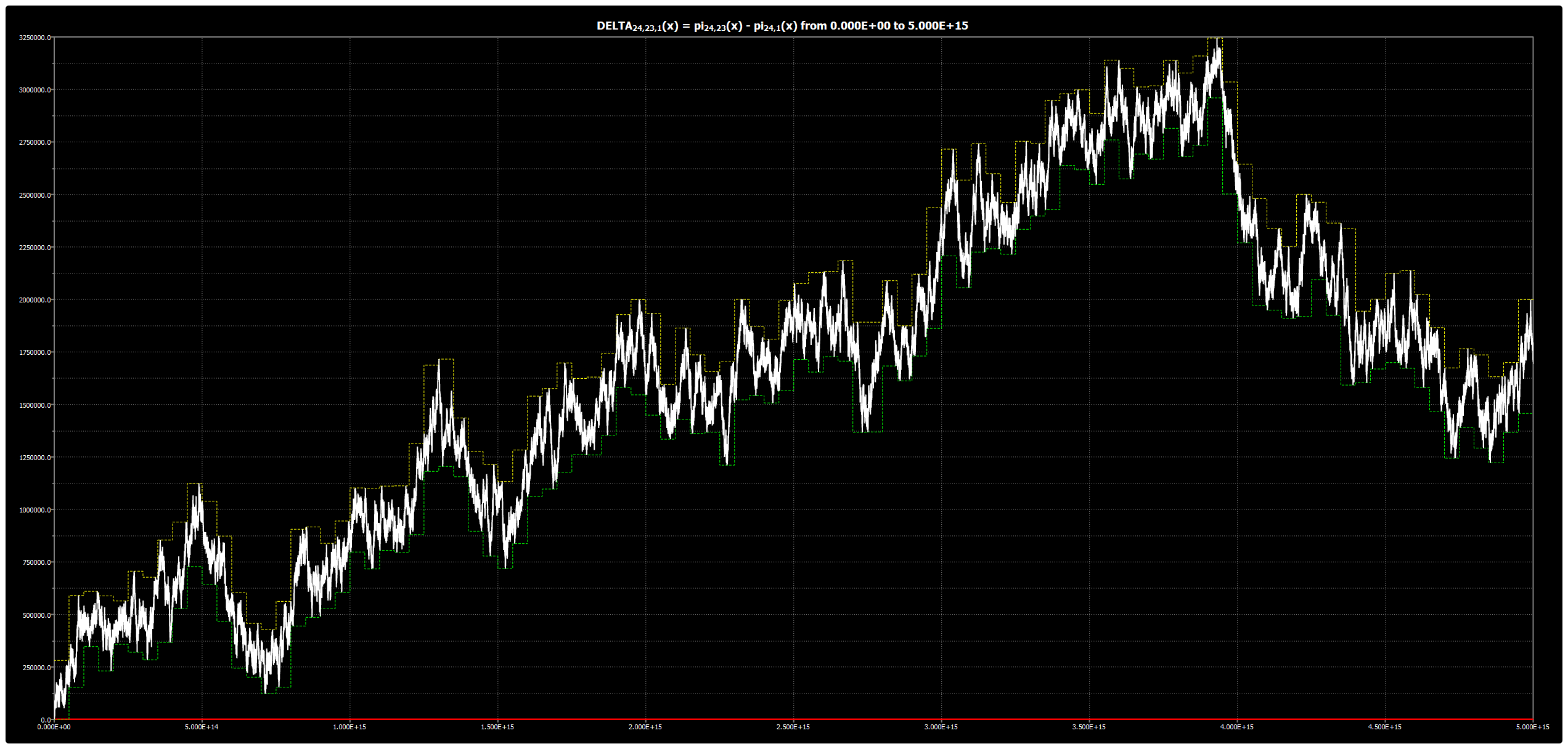|

# Chebyshev’s Bias: Chart for DELTA{24,19,1}(x)

We are publishing the chart for DELTA{24,19,1}(x) up to 5*1015 in Chebyshev’s Bias. The chart was obtained using our Chebyshev’s Bias Visualizer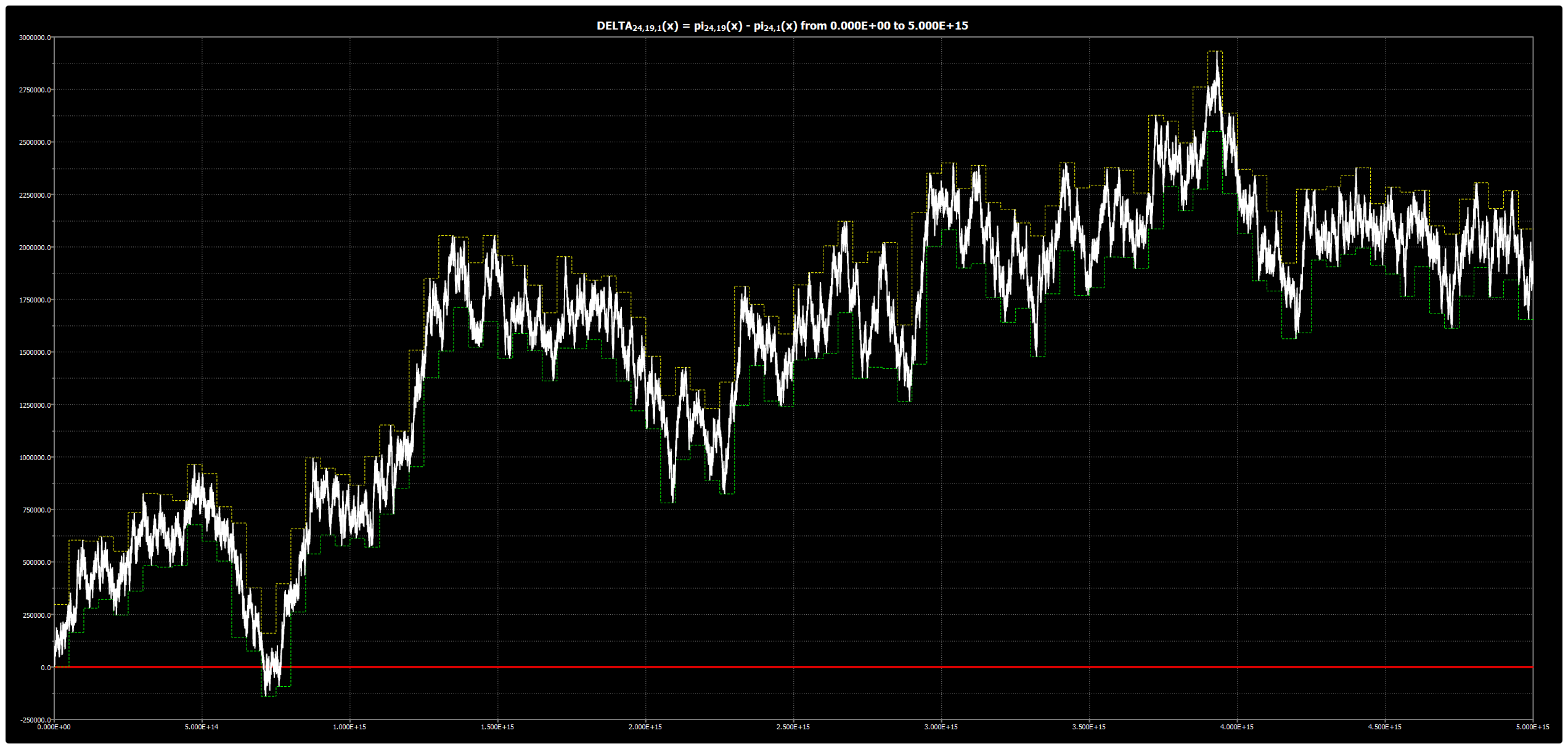|

# Chebyshev’s Bias: Chart for DELTA{24,17,1}(x)

We are publishing the chart for DELTA{24,17,1}(x) up to 5*1015 in Chebyshev’s Bias. The chart was obtained using our Chebyshev’s Bias Visualizer.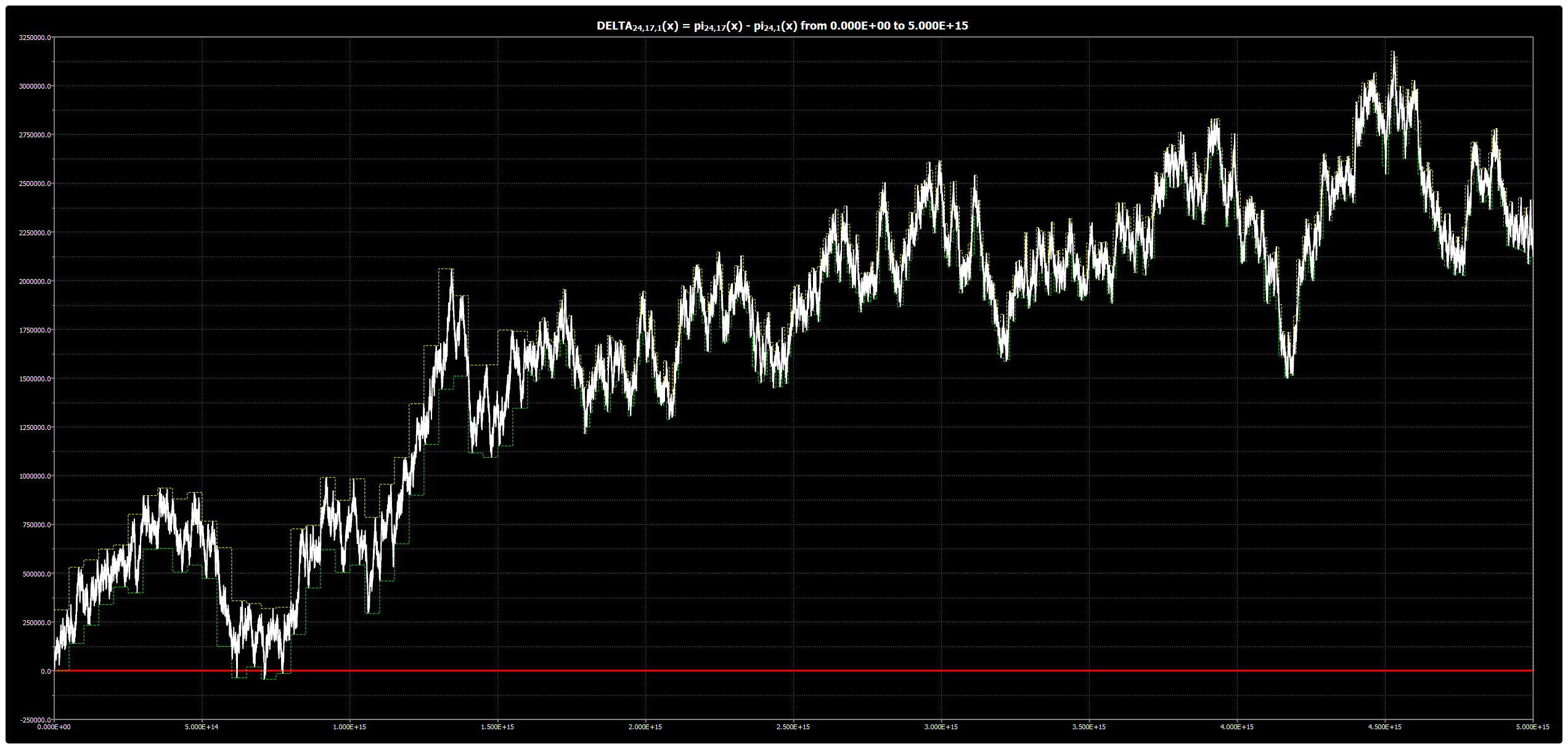|

# Chebyshev’s Bias: Chart for DELTA{24,13,1}(x)

We are publishing the chart for DELTA{24,13,1}(x) up to 5*1015 in Chebyshev’s Bias. The chart was obtained using our Chebyshev’s Bias Visualizer.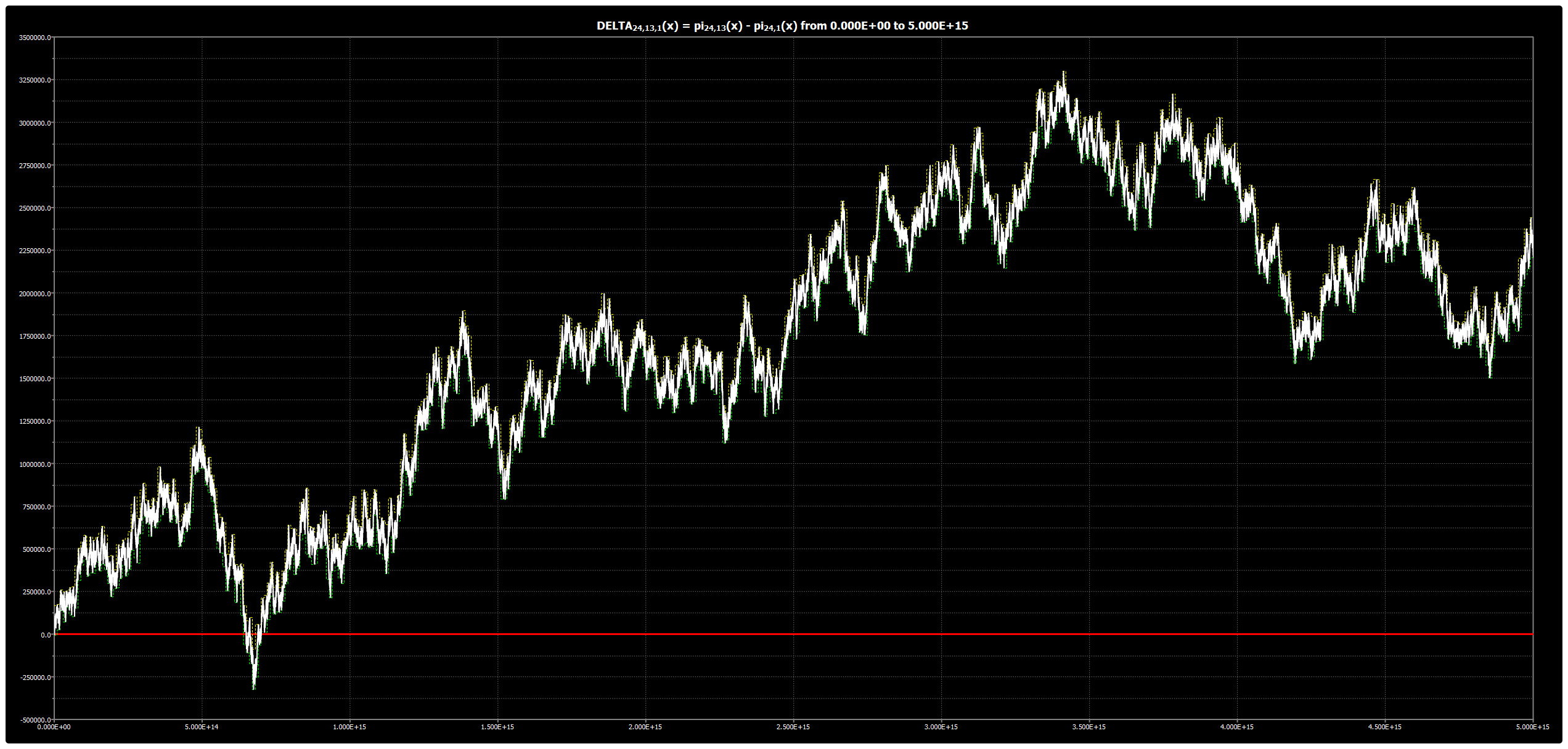|

# Chebyshev’s Bias: Chart for DELTA{24,11,1}(x)

We are publishing the chart for DELTA{24,11,1}(x) up to 5*1015 in Chebyshev’s Bias. The chart was obtained using our Chebyshev’s Bias Visualizer.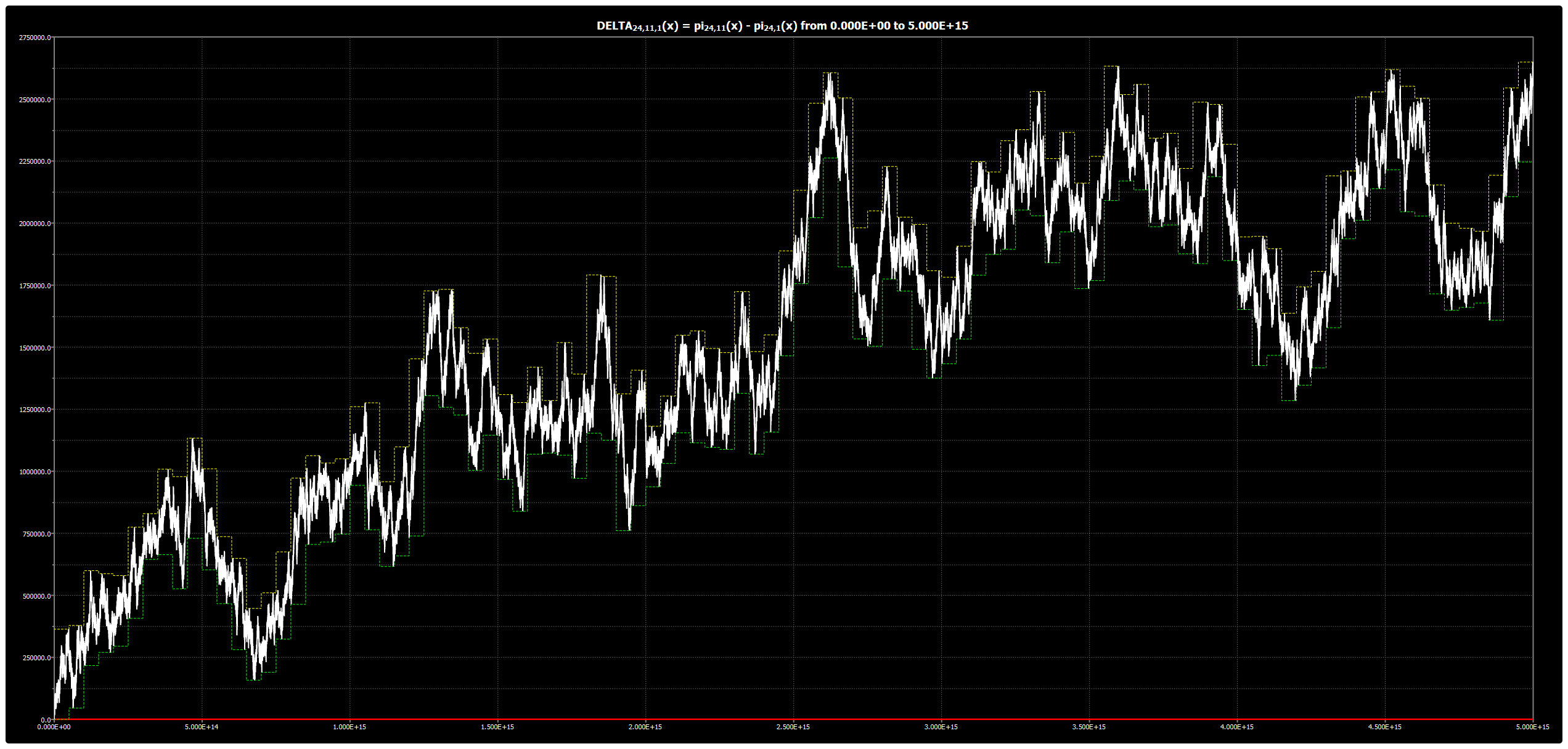|

# Chebyshev’s Bias: Chart for DELTA{24,7,1}(x)

We are publishing the chart for DELTA{24,7,1}(x) up to 5*1015 in Chebyshev’s Bias. The chart was obtained using our Chebyshev’s Bias Visualizer.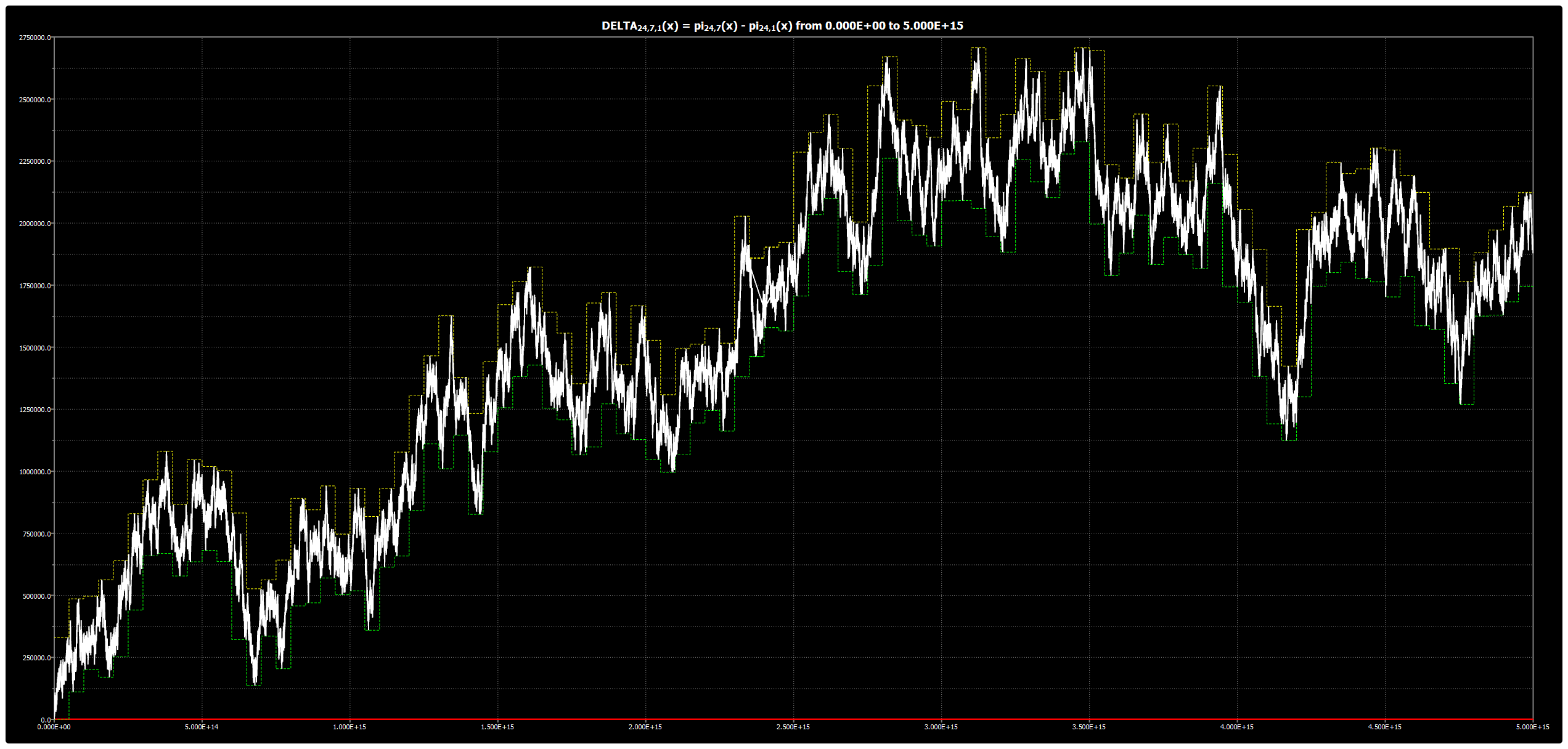|

# Chebyshev’s Bias: Chart for DELTA{24,5,1}(x)

We are publishing the chart for DELTA{24,5,1}(x) up to 5*1015 in Chebyshev’s Bias. The chart was obtained using our Chebyshev’s Bias Visualizer.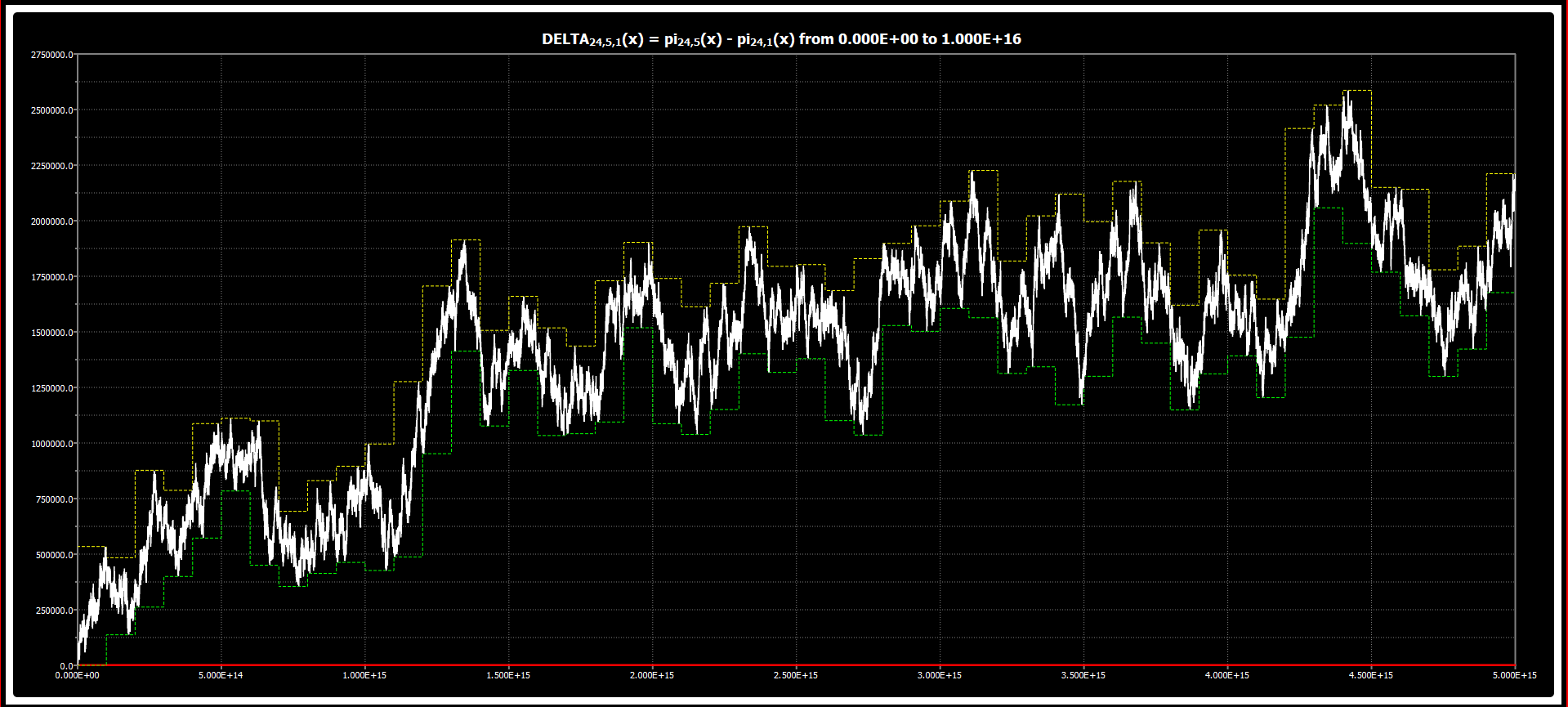|

# Chebyshev’s Bias: Chart for DELTA{12,11,1}(x)

We are publishing the chart for DELTA{12,11,1}(x) up to 5*1015 in Chebyshev’s Bias. The chart was obtained using our Chebyshev’s Bias Visualizer.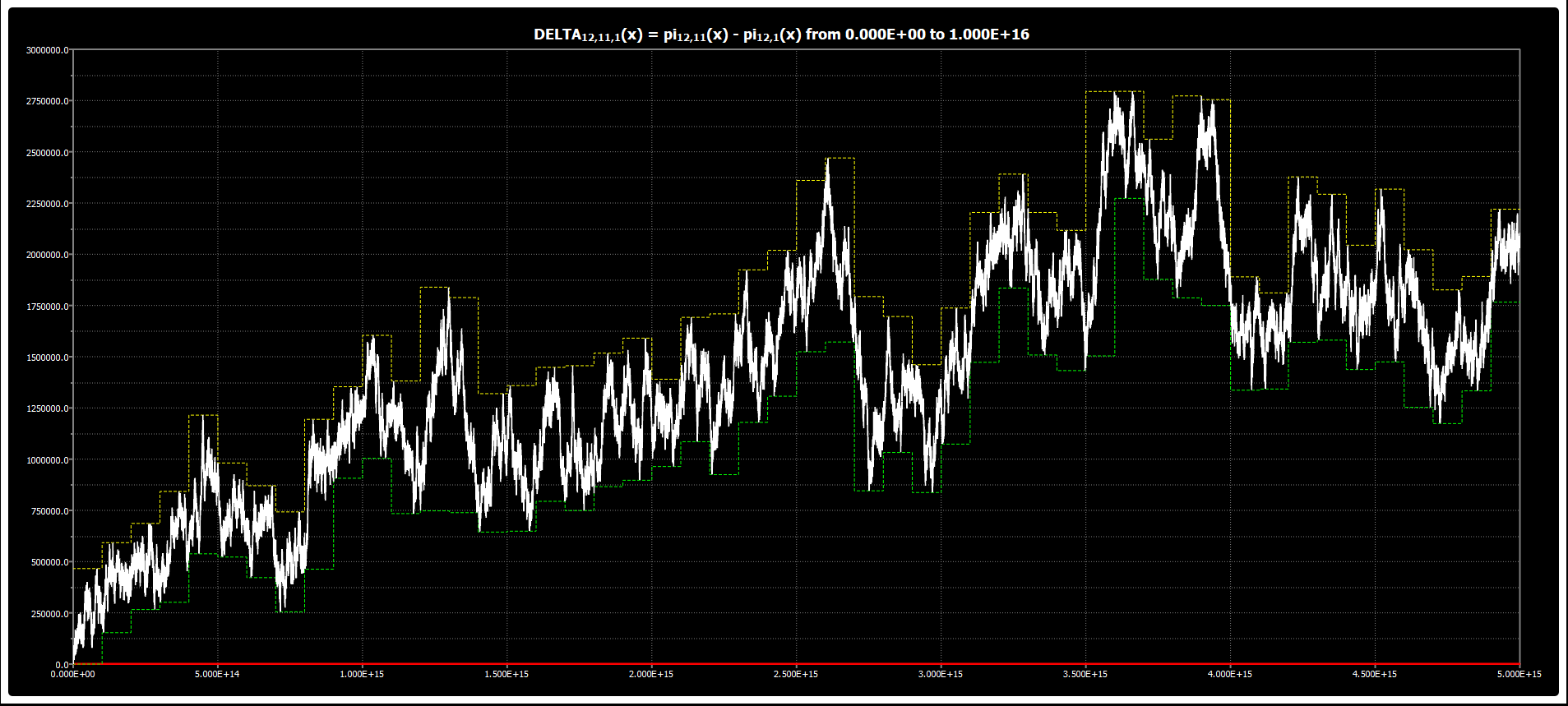|

# Chebyshev’s Bias: Chart for DELTA{12,7,1}(x)

We are publishing the chart for DELTA{12,7,1}(x) up to 5*1015 in Chebyshev’s Bias. The chart was obtained using our Chebyshev’s Bias Visualizer.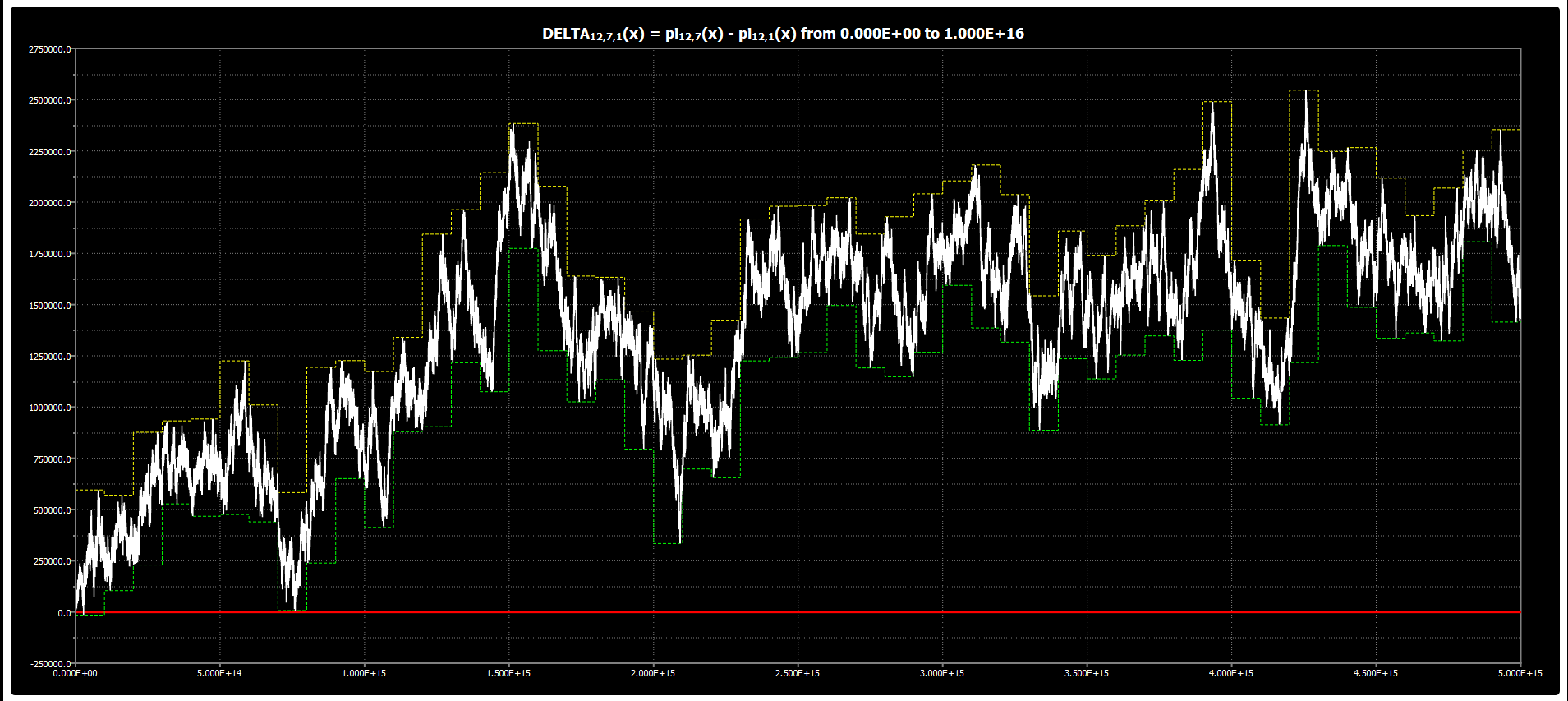|

# Chebyshev’s Bias: Chart for DELTA{12,5,1}(x)

We are publishing the chart for DELTA{12,5,1}(x) up to 5*1015 in Chebyshev’s Bias. The chart was obtained using our Chebyshev’s Bias Visualizer.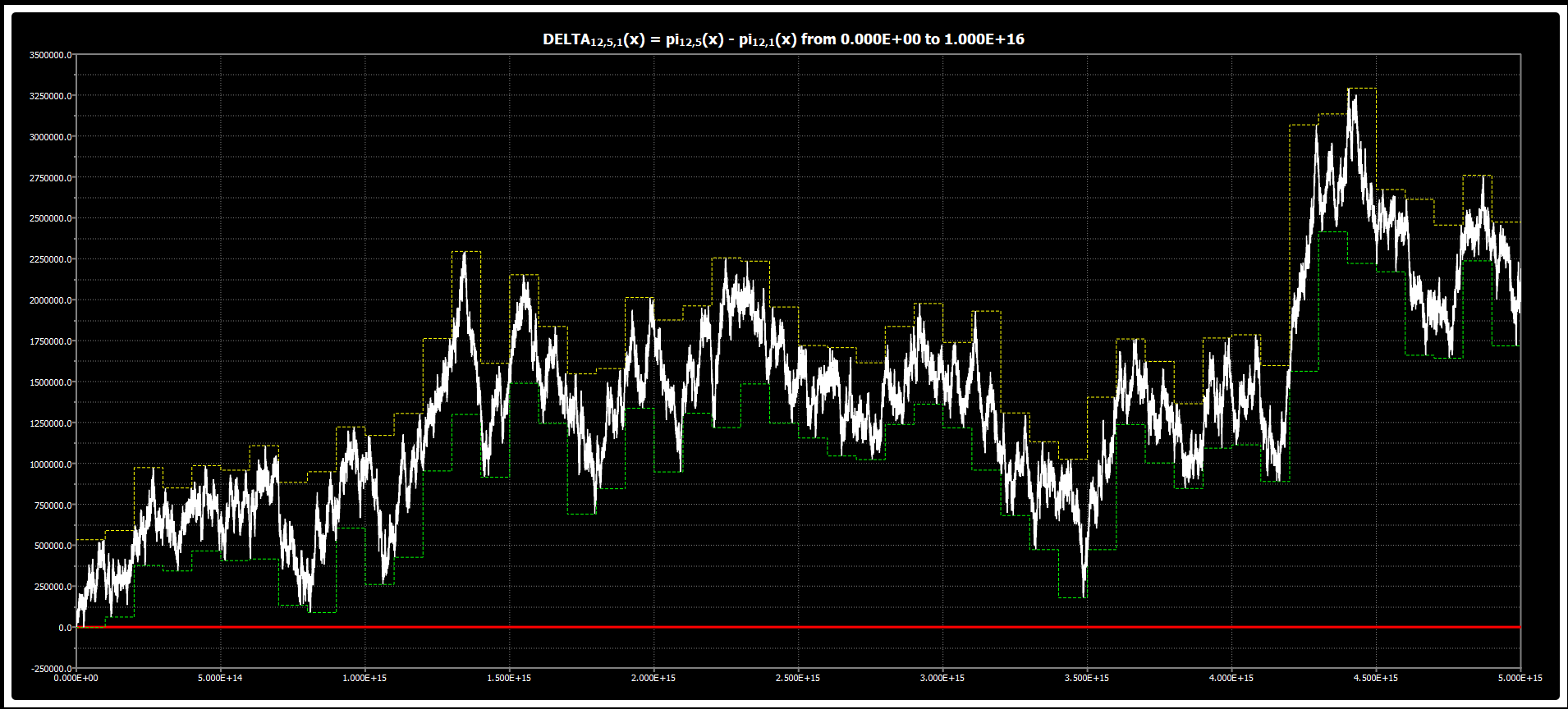|

# Chebyshev’s Bias: Chart for DELTA{8,7,1}(x)

We are publishing the chart for DELTA{8,7,1}(x) up to 5*1015 in Chebyshev’s Bias. The chart was obtained using our Chebyshev’s Bias Visualizer.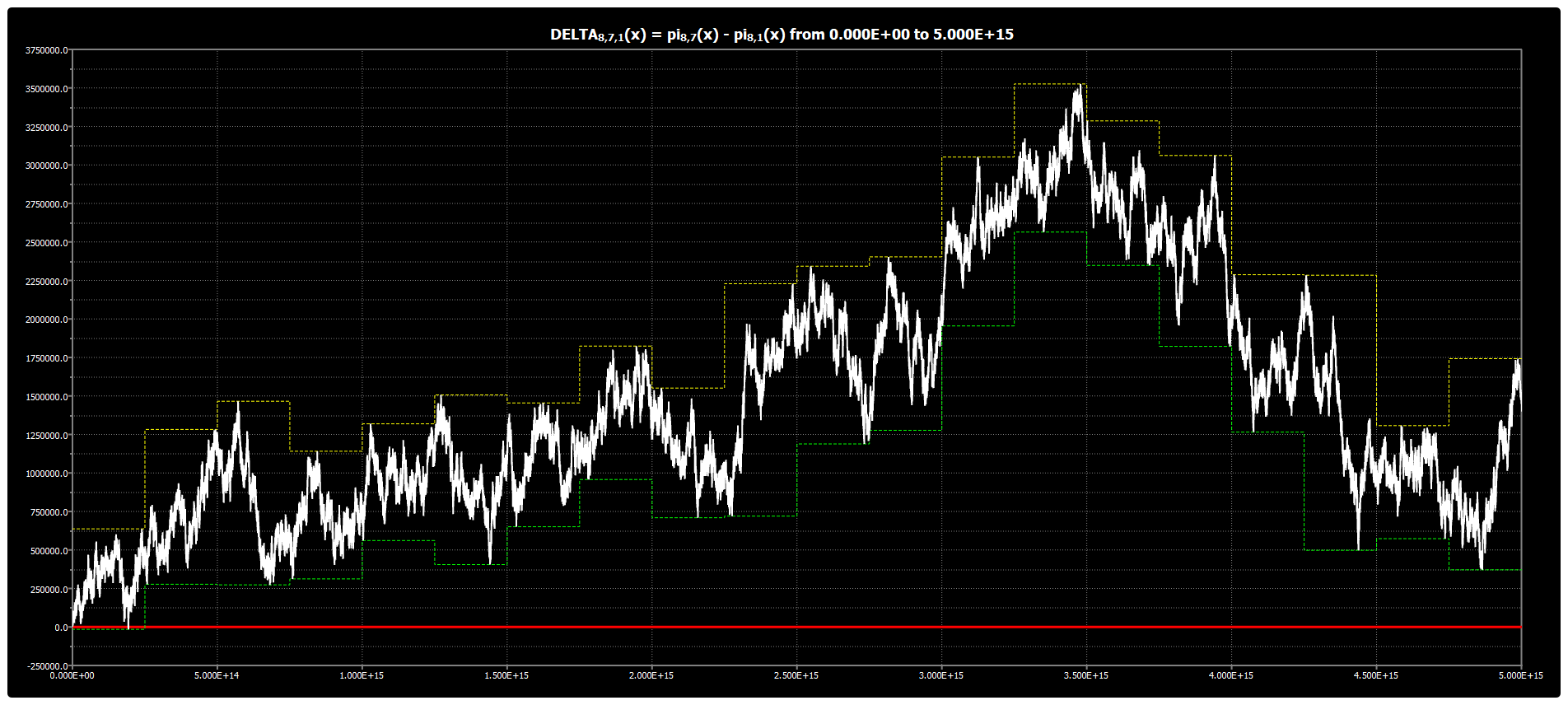|

# Chebyshev’s Bias: Chart for DELTA{8,5,1}(x)

We are publishing the chart for DELTA{8,5,1}(x) up to 5*1015 in Chebyshev’s Bias. The chart was obtained using our Chebyshev’s Bias Visualizer.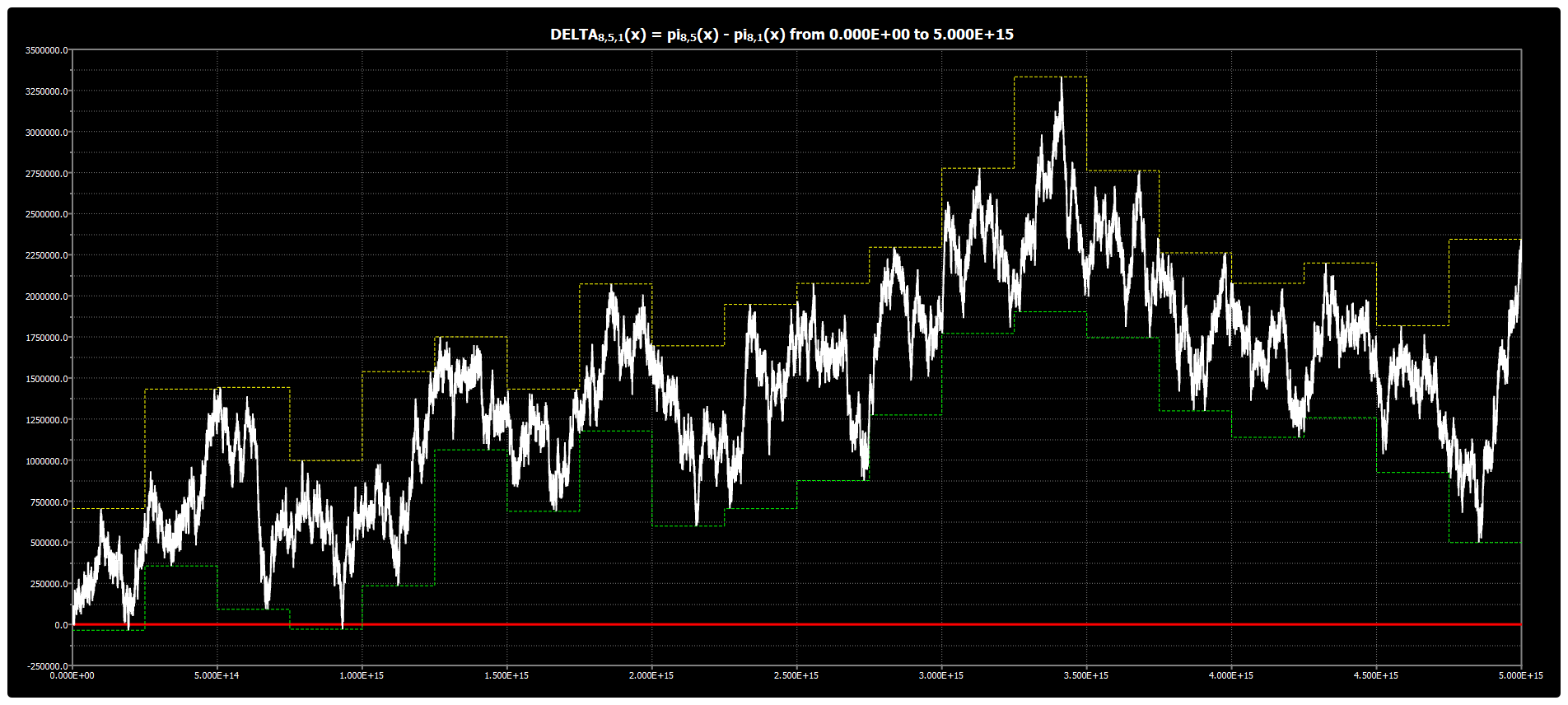|

# Chebyshev’s Bias: Chart for DELTA{8,3,1}(x)

We are publishing the chart for DELTA{8,3,1}(x) up to 5*1015 in Chebyshev’s Bias. The chart was obtained using our Chebyshev’s Bias Visualizer.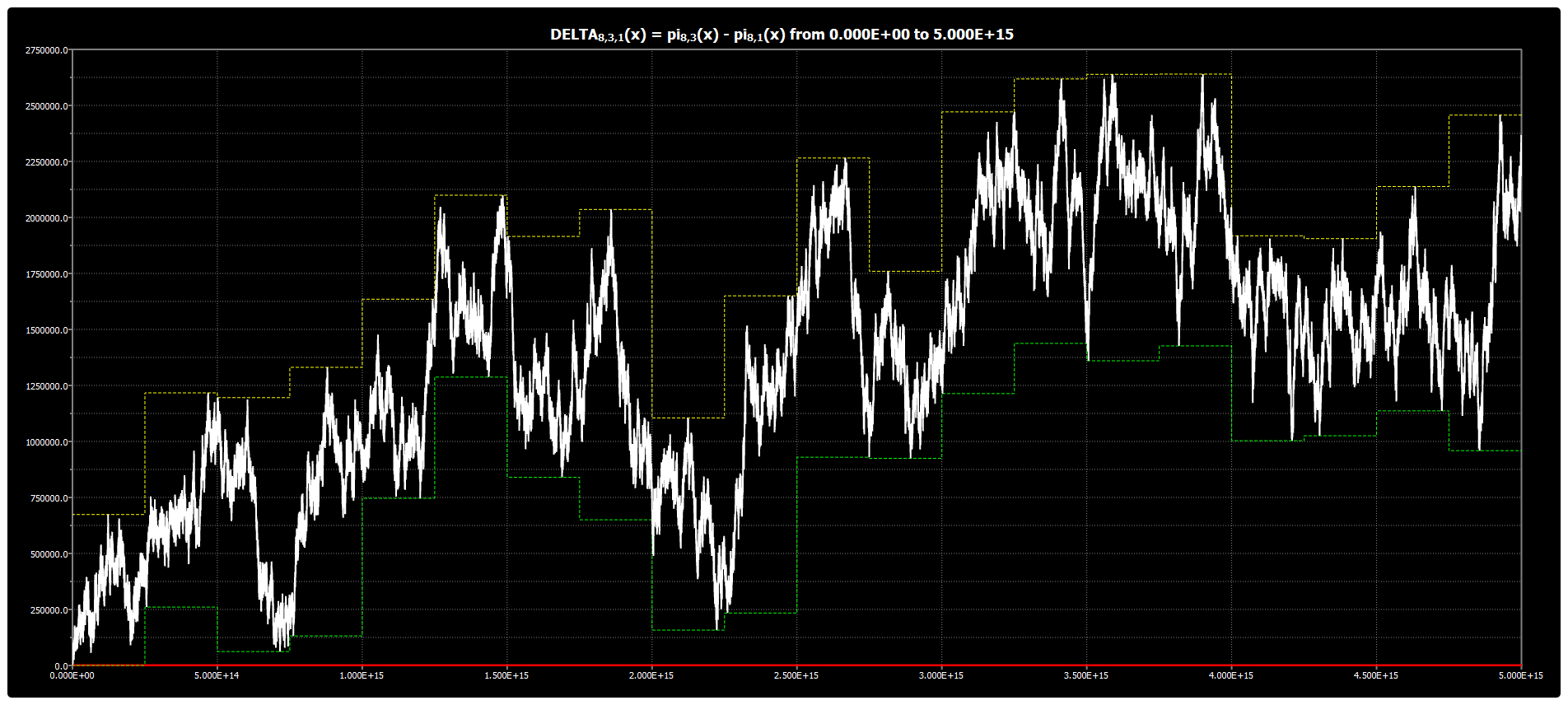|

# Chebyshev’s Bias: Chart for DELTA{3,2,1}(x)

We are publishing the chart for DELTA{3,2,1}(x) up to 5*1015 in Chebyshev’s Bias. The chart was obtained using our Chebyshev’s Bias Visualizer.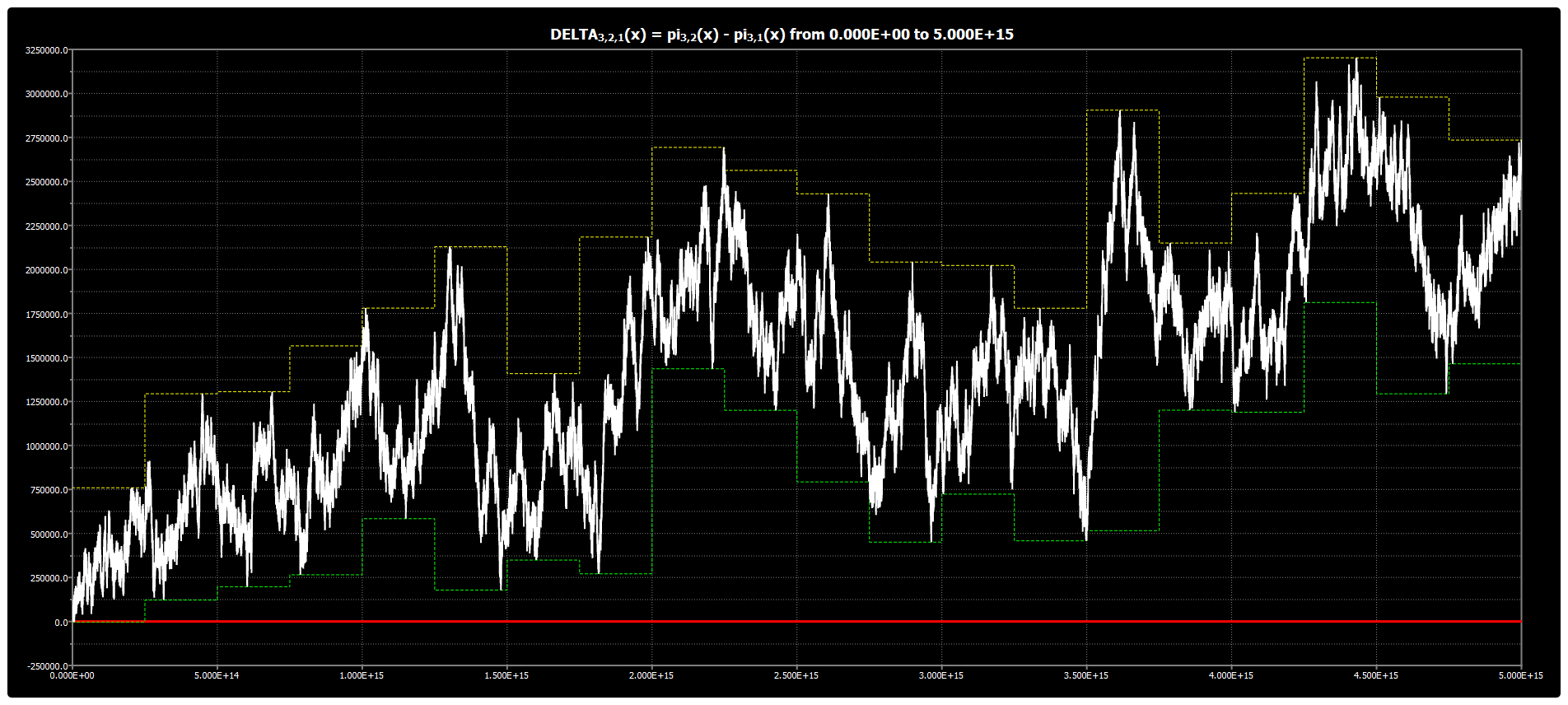|

# MacOS version of new “Chebyshev’s Bias Visualizer” released

We are releasing the MacOS version for the new “Chebyshev’s Bias Visualizer”. The new version has an improved user interface as well as a number of additional features like chart scrolling and zooming. For your convenience, the executable is released in .app as well as in .dmg versions.